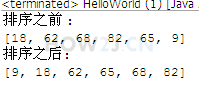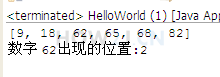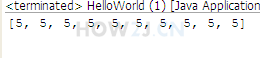# 数组系列教材 - java.util.Arrays类常用方法

2022年4月18日 59点热度 0人点赞 0条评论

Arrays是针对数组的工具类，可以进行 排序，查找，复制填充等功能。 大大提高了开发人员的工作效率。

## 步骤 1 : 数组复制

 `import` `java.util.Arrays;`   `public` `class` `HelloWorld {` `    ``public` `static` `void` `main(String[] args) {` `        ``int` `a[] = ``new` `int``[] { ``18``, ``62``, ``68``, ``82``, ``65``, ``9` `};`   `        ``// copyOfRange(int[] original, int from, int to)` `        ``// 第一个参数表示源数组` `        ``// 第二个参数表示开始位置(取得到)` `        ``// 第三个参数表示结束位置(取不到)` `        ``int``[] b = Arrays.copyOfRange(a, ``0``, ``3``);`   `        ``for` `(``int` `i = ``0``; i < b.length; i++) {` `            ``System.out.print(b[i] + ``" "``);` `        ``}`   `    ``}` `}`

## 步骤 2 : 转换为字符串

 `import` `java.util.Arrays;` ` `  `public` `class` `HelloWorld {` `    ``public` `static` `void` `main(String[] args) {` `        ``int` `a[] = ``new` `int``[] { ``18``, ``62``, ``68``, ``82``, ``65``, ``9` `};` `        ``String content = Arrays.toString(a);` `        ``System.out.println(content);` ` `  `    ``}` `}`

## 步骤 3 : 排序`import` `java.util.Arrays;` ` `  `public` `class` `HelloWorld {` `    ``public` `static` `void` `main(String[] args) {` `        ``int` `a[] = ``new` `int``[] { ``18``, ``62``, ``68``, ``82``, ``65``, ``9` `};` `        ``System.out.println(``"排序之前 :"``);` `        ``System.out.println(Arrays.toString(a));` `        ``Arrays.sort(a);` `        ``System.out.println(``"排序之后:"``);` `        ``System.out.println(Arrays.toString(a));` ` `  `    ``}` `}`

## 步骤 4 : 搜索`import` `java.util.Arrays;`   `public` `class` `HelloWorld {` `    ``public` `static` `void` `main(String[] args) {` `        ``int` `a[] = ``new` `int``[] { ``18``, ``62``, ``68``, ``82``, ``65``, ``9` `};`   `        ``Arrays.sort(a);`   `        ``System.out.println(Arrays.toString(a));` `        ``//使用binarySearch之前，必须先使用sort进行排序` `        ``System.out.println(``"数字 62出现的位置:"``+Arrays.binarySearch(a, ``62``));` `    ``}` `}`

## 步骤 5 : 判断是否相同

 `import` `java.util.Arrays;`   `public` `class` `HelloWorld {` `    ``public` `static` `void` `main(String[] args) {` `        ``int` `a[] = ``new` `int``[] { ``18``, ``62``, ``68``, ``82``, ``65``, ``9` `};` `        ``int` `b[] = ``new` `int``[] { ``18``, ``62``, ``68``, ``82``, ``65``, ``8` `};`   `        ``System.out.println(Arrays.equals(a, b));` `    ``}` `}`

## 步骤 6 : 填充`import` `java.util.Arrays;` ` `  `public` `class` `HelloWorld {` `    ``public` `static` `void` `main(String[] args) {` `        ``int` `a[] = ``new` `int``[``10``];` ` `  `        ``Arrays.fill(a, ``5``);` ` `  `        ``System.out.println(Arrays.toString(a));` ` `  `    ``}` `}`zdc1228@163.com